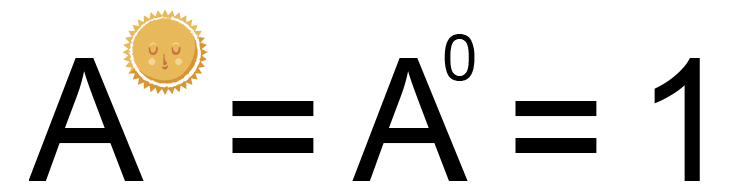# Indices law - Law 4

## a^0=1

How to remember a^0=1

Anything to the power zero is 1It doesn't matter how many people there are there is only one sun.One sun is one.

## But why?

Try simple numbers you know first

We know 10/10=1

But so too 10^2/10^2=1

But this can be rewritten as  10^2\times 10^-2

And rewritten again as 10^(2-2)=10^0

So 10^0 must be 1